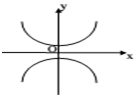If the two curves in the figure given alongside have the magnitudes of their respective minimum and maximum values as equal, which of the following pair of equations may represent the two curves?